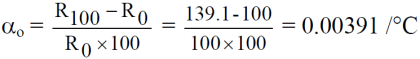# Calculate Temperature Coefficient of RTD

RTD’s are based on the principle that the resistance of a metal increases with temperature. The temperature coefficient of resistance (TCR) for resistance temperature detectors (denoted by αo), is normally defined as the average resistance change per °C over the range 0 °C to 100 °C, divided by the resistance of the RTD, Ro, at 0 °C.where,

R0 = resistance of rtd at 0 °C (ohm), and

R100 = resistance of rtd at 100 °C (ohm),

Note: Here we are discussing about RTD PT100 only.As a first approximation, the relationship between resistance and temperature, may then be expressed as (see Figure 2):where: Rt = resistance of rtd at temperature t (ohm),

Ro = resistance of rtd at 0 °C (ohm), and

α= temperature coefficient of resistance (TCR) at 0 °C (per °C)

Example

#### Calculate the Temperature Coefficient of RTD PT100

From Equation – 1 :#### Calculate the resistance of the RTD at 50 °C

From Equation – 2 :

R50 = Ro(1 + αt) = 100(1 + 0.00391×50) = 119.55Ω

#### Calculate the temperature when the resistance is 110 ohms

From Equation – 2:

Rt = Ro(1 + αt) ⇒ 110 = 100(1 + 0.00391t)

Rt =1 + 0.00391t = 1.1 ⇒ 0.00391t = 0.1 ⇒ t = 25.58 °C.

Also Read : RTD Working Principle

Be the first to get exclusive content straight to your email.
We promise not to spam you. You can unsubscribe at any time.

### 8 thoughts on “Calculate Temperature Coefficient of RTD”

1.Dear sir,
last question u made a mistake that calculate the temperature for the resistance at 110 ohm but u mentioned 100 *c

•Thank you Anand. updated the answer.

•please sir help me in this calculation,
the resistance Rt of a platinum wire at temperature Tdegree measured on the glass scale is given by Rt=Ro(1 + at +bt) where a=3.800*10^MINUS3 and b=5.6*10^minus7 .What temperature will the platinum thermometer indicate when the temperature of the gas scale is
200degree

2.I think there is another easy method to convert ohms to temperature
To convert temperature to ohms..
If u knows then write please

3.subtract the ohms you get from 138.5 and the answer you get divide by 38.5 then multiply by 100. eg. 110ohms from 138.5 ohms you get 28.5 ohms. divide that by 38.5 equals 0.714 multiply by 100 equals 71.2 deg.

4.thank you

5.Some of the time we use 2.5 to multiply the RF

How can we prove that 2.5 as the standard unit
And also we did we get that 2.5 from

6.